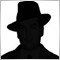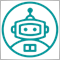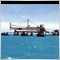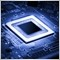• Overview
• Reviews (7)

# ImpAO

The Awesome Oscillator (AO) indicator is calculated by median price using simple Moving Averages with periods 5 and 34.

This modification allows calculating the indicator on the basis of any combination of 4 basic prices: Close, Open, High, and Low, select periods and the method of the Moving Average: simple (SMA), exponential (EMA), smoothed (SMMA), linear weighted (LWMA), double exponential (DEMA), triple exponential (TEMA).

Input parameters:

• Fast period - a period of fast Moving Average;
• Slow period - a period of slow Moving Average;
• Moving Average mode - the averaging method: Simple (SMA), Exponential (EMA), Smoothed (SMMA), Linear Weighted (LWMA), Double Exponential (DEMA), Triple Exponential (TEMA).
• Close - weight coefficient of CLOSE price for calculation of the individual price;
• Open - weight coefficient of OPEN price for calculation of the individual price;
• High - weight coefficient of HIGH price for calculation of the individual price;
• Low - weight coefficient of LOW price for calculation of the individual price.

Example 1. Calculate the indicator by OPEN price: Close=0, Open=1, High=0, Low=0.
Example 2. Calculate the indicator by WEIGHTED price: Close=2, Open=0, High=1, Low=1.
Example 3. Calculate the indicator by individual price constant: Close=6, Open=7, High=1, Low=3. Price=(6*Close + 7*Open + 1*High + 3*Low)/17.16101
Nikolay Gaylis 2019.01.14 08:21

User didn't leave any comment to the rating12010
2018.02.01 17:30

User didn't leave any comment to the rating5698
2017.11.09 14:48

User didn't leave any comment to the rating6207
2017.07.21 17:34

User didn't leave any comment to the rating7183
2016.03.14 04:27

User didn't leave any comment to the rating7827
2014.04.04 09:46

User didn't leave any comment to the rating109
Jenny 2014.02.25 14:30

норм